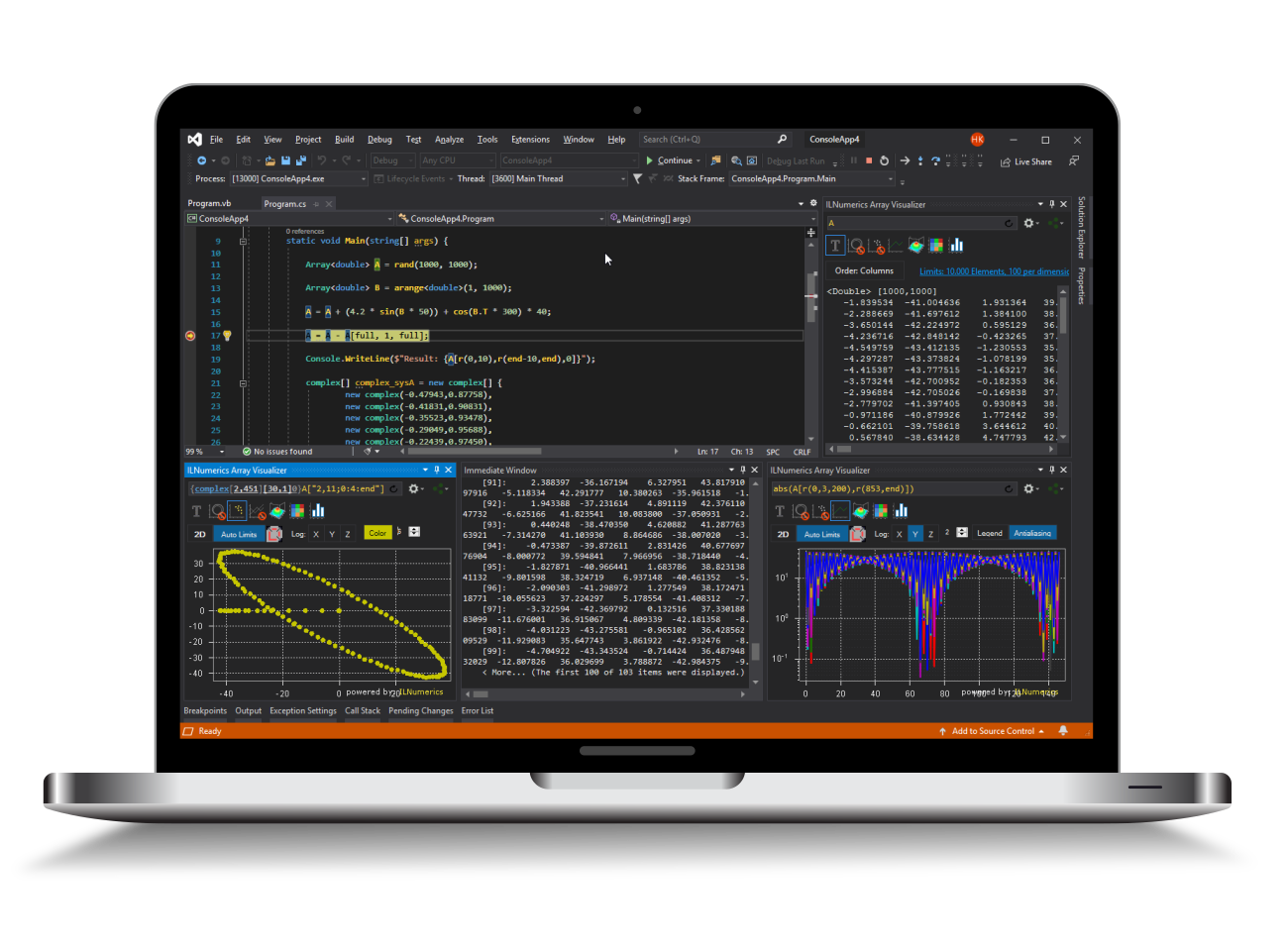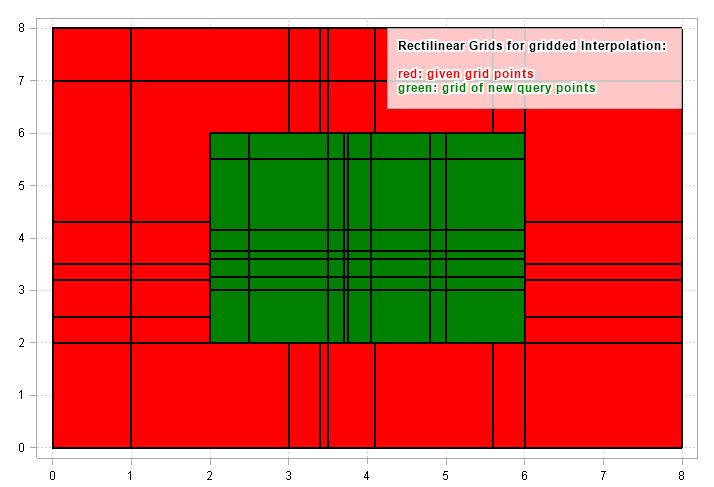Industrial Data Science
in C# and .NET:
Simple. Fast. Reliable.ILNumerics - Technical Computing

Modern High Performance Tools for Technical

Computing and Visualization in Industry and Science

tgt

# Interpolating Grids from Gridded Data

This sections deals with the interpolation of new data from an existing grid of known data points and the special case, that the new data points are ordered in a grid themself. Talking about grids implies that the data are measured in $R^2\dots R^n$. One dimensional data are handled in their own article.

## Overview

Both, the source data grid and the grid of new interpolated points are rectilinear. However, both grids are not required to be regular, i.e.: the spacing between adjacent grid elements may vary within each dimension.

One example of a rectilinear grid is given in the following image:Here, the known source samples were acquired at the grid crossings of the red areas. All tiles formed by the grid lines are rectangles: they show all angles of 90° only. However, these rectangles are not necessarily congruent, as can clearly be seen from the image above.

The green grid tiles above are an example of a gridded set of interpolating query points. The same is true here as for the known samples: the spacing between the grid lines is not required to be equal - not within one dimension and not among the dimensions.

It must be noted that regular grids with equal spacings are a special case of the general non-regular grids and applicable for the methods in this section, of course.

## Functions for Gridded Data Interpolation on Grids

The following functions allow the interpolation of gridded data. They are all found in the ILNumerics Interpolation Toolbox as public static members of the ILNumerics.Toolboxes.Interpolation class:

Function Description Dimension
interp2() All interpolation methods on 2-dimensional data. Dimension order is expected in meshgrid format (rows first).
interp3() All interpolation methods on 3-dimensional data. Dimension order is expected in meshgrid format (rows first).
interpn() All interpolation methods on n-dimensional data. Dimension order is expected in natural format (columns first).
Specialized interpolation methods
splinen() Spline interpolation methods on n-dimensional data. See here. Dimension order is expected in natural format (columns first).

All functions are specifically designed for multidimensional data. General interpolation is performed by the interp2, interp3, and interpn interface. This gives access to all grid interpolation methods: linear, nearest-/next-/previous neighbor, polynomial, piecewise cubic and spline interpolation. For multidimensional gridded spline interpolation with non-standard parameters a specialized interface splinen() is provided and described in the article on spline interpolation.

In the following paragraphs the interp?() functions are described.

## Interpolation of 2-dimensional Grids: interp2()

The interp2() function creates a 2D grid of newly interpolated values from a 2D grid of known values. Both grids can arbitrarily be defined by the parameters X1, X2 and Xn1,Xn2 respectively. The function allows the definition of the interpolation method and, optionally, of the extrapolation behavior.

Example: Create a finer grid of data sampled from a 2-dimensional function.

The interpolation is performed in the line:

The following data are given to the interp2() function:

• V - the matrix of data values,
• X1 and X2 - define the grid positions (coordinates) of the known points,
• Xn1 and Xn2 - define the query point grid to be newly interpolated, and
• method - the interpolation method.

### Definition of known Points

The matrix V in the example above stores the values of the known points. The positions for the known points are defined by the X1 and X2 variables. Since the points are expected to be ordered as a grid, it is sufficient to define the coordinates of the vertical and horizontal grid lines as vectors of positions in X1 and X2. Those vectors must contain strictly monotonically increasing elements.

Alternatively, X1 and X2 can be matrices of the same size as V. In this case, the value, as well as the x and y coordinates of a known point are found at matching elements in all matrices: V, X1 and X2.

As a third alternative, X1 or X2 can be provided as null which will lead to the auto-generation of the position vectors: starting at 0, step size 1.0 over the length of the corresponding dimension in V.

### Order of Dimensions

An important distinction is made for the order of the dimensions in V: interp2() and interp3() are optimized for plotting purposes. I.e.: they expect the first dimension to go along the rows, the second dimension to go along the columns. This corresponds to the common look and feel of most popular plots: the x axis runs horizontally while the y axis goes vertically. In ILNumerics and many other similar systems, this order is associated with the output of the meshgrid() function:

Note, that the vector of grid points given as X parameter is reflected in the output along the rows. Similarly, the Y values starting at -10 go along the columns as can be seen from the 2nd output of meshgrid:

Keep in mind that it is only interp2() and interp3() which expose this ordering of dimensions. interpn() and any other gridded function expects the dimensions of V in their 'natural' format, i.e.: the first dimension runs along the columns, the second dimension is associated with the rows.

Definition of new Query Points

The grid of newly interpolated points is defined by the grid vectors Xn. The same rules apply for Xn as for the definition of the grid of known values X:

• Xn1 or Xn2 can be null. In this case new query points are derived by subdividing the corresponding ranges of X. Only therefore the k parameter is taken into account.
• Xn1 and Xn2 can be vectors of the length of the corresponding dimension in V, with strictly monotonically increasing elements.
• Or, any of Xn1 or Xn2 can be the result of applying above grid vectors to the meshgrid() function.

### Optional Parameters

Most of the parameters are optional and can be omitted. In the most simple case, only V is given and X as well as Xn are automatically generated for you:

How fine the grid generated will be, can also be controlled via the k parameter:

### Extrapolation, Out-of-Range Values

By default, interp2() assigns NaN to any new query point laying outside of the range defined for V. This behavior can be controlled by utilizing the overload of interp2() which provides the outOfRangeValues parameter. Here come the options:

• Set this value to a numerical constant or NaN. This constant will be assigned to all out-of-range values. Note that setting this value to NaN leads to the same result than the utilization of the interp2() overload without the outOfRangeValues parameter.
• Alternatively, if null is given for the outOfRangeValues parameter, values for out-of-range query points are computed by extrapolation from known query points using the selected interpolation method.

Example: Linear extrapolation on a default 2D grid

The following two calls are equivalent and produce NaN values at out-of-range points:

## Interpolation of 3-dimensional Grids: interp3()

In the 3-dimensional case the interp3() function can be used in a very similar way. The function exposes a third parameter for defining the grid positions X3 of the known values and the parameter Xn3 for the new query point positions. All other parameters and options are similarly applicable.

## Interpolation of n-dimensional Grids: interpn()

In the general case of n-dimensional data V the interface of Interpolation.interpn() must allow the definition of arbitrary numbers of dimensions. Therefore, X? and Xn? are wrapped into a cell array, allowing to provide multiple grid definitions at once. However, the general scheme of providing grid position data X for the known values V and grid positions Xn for new query points stay the same.

Example: 3-dimensional piecewise cubic interpolation and extrapolation.

Keep the following notes in mind:

• The dimensionality of the problem is determined by the number of dimensions in V.
• The order of dimensions for interpn() differs from interp2() and interp3(): the first dimension is associated with the columnn in V, the second dimension with the rows.
• X and Xn can define the grid positions for any dimension in V. Any dimension not defined is handled with the same default rules as for interp2.
• All interpolation methods from the enum InterpolationMethod are applicable.

Refer to the apidoc class reference documentation for interpn() for more details.

## A Note on Performance

All interpolation methods in this section (as so for all in ILNumerics Interpolation Toolbox) are carefully hand tuned for best performance and smallest resource consumption. They parallelize on multicore systems and are well prepared to be used on large data sets and in low latency scenarios.

See also: